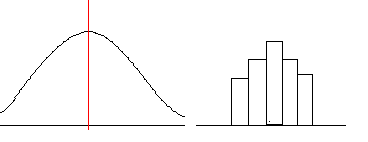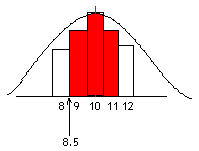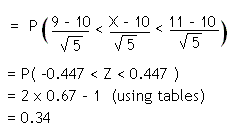## Normal Approximations

Binomial Approximation

The normal distribution can be used as an approximation to the binomial distribution, under certain circumstances, namely:

• If X ~ B(n, p) and if n is large and/or p is close to ½, then X is approximately N(np, npq)

(where q = 1 - p).

In some cases, working out a problem using the Normal distribution may be easier than using a Binomial.

Poisson Approximation

The normal distribution can also be used to approximate the Poisson distribution for large values of l (the mean of the Poisson distribution).

• If X ~ Po(l) then for large values of l, X ~ N(l, l) approximately.

Continuity Correction

The binomial and Poisson distributions are discrete random variables, whereas the normal distribution is continuous. We need to take this into account when we are using the normal distribution to approximate a binomial or Poisson using a continuity correction.

In the discrete distribution, each probability is represented by a rectangle (right hand diagram):When working out probabilities, we want to include whole rectangles, which is what continuity correction is all about.

Example

Suppose we toss a fair coin 20 times. What is the probability of getting between 9 and 11 heads?

Let X be the random variable representing the number of heads thrown.
X ~ Bin(20, ½)

Since p is close to ½ (it equals ½!), we can use the normal approximation to the binomial. X ~ N(20 × ½, 20 × ½ × ½) so X ~ N(10, 5) .

In this diagram, the rectangles represent the binomial distribution and the curve is the normal distribution:We want P(9 ≤ X ≤ 11), which is the red shaded area. Notice that the first rectangle starts at 8.5 and the last rectangle ends at 11.5 . Using a continuity correction, therefore, our probability becomes P(8.5 < X < 11.5) in the normal distribution.

Standardising